Fast Fourier Transform

As shown in the last subsection, kernel density estimates can be expressed as a submatrix of a certain convolution. The fast Fourier transform (FFT) is a computationally effective method for computing such convolutions. For a reference on this material, see Press et al. (1988).

The discrete Fourier transform of a complex vector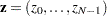is the vector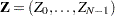, where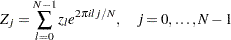andis the square root of
. The vectorcan be recovered from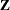by applying the inverse discrete Fourier transform formula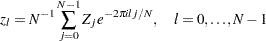Discrete Fourier transforms and their inverses can be computed quickly using the FFT algorithm, especially when
is highly composite; that is, it can be decomposed into many factors, such as a power of. By the discrete convolution theorem, the convolution of two vectors is the inverse Fourier transform of the element-by-element product of their Fourier transforms. This, however, requires certain periodicity assumptions, which explains why the vectors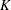and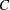require zero-padding. This is to avoid wrap-around effects (refer to Press et al.; 1988, pp. 410–411). The vectoris actually mirror-imaged so that the convolution ofandwill be the vector of binned estimates. Thus, if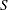denotes the inverse Fourier transform of the element-by-element product of the Fourier transforms ofand, then the firstelements ofare the estimates.

The bivariate Fourier transform of an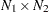complex matrix having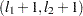entry equal to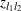is thematrix with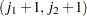entry given by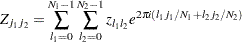and the formula of the inverse is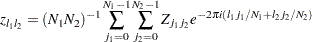The same discrete convolution theorem applies, and zero-padding is needed for matricesand. In the case of, the matrix is mirror-imaged twice. Thus, ifdenotes the inverse Fourier transform of the element-by-element product of the Fourier transforms ofand, then the upper-left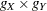corner ofcontains the estimates.